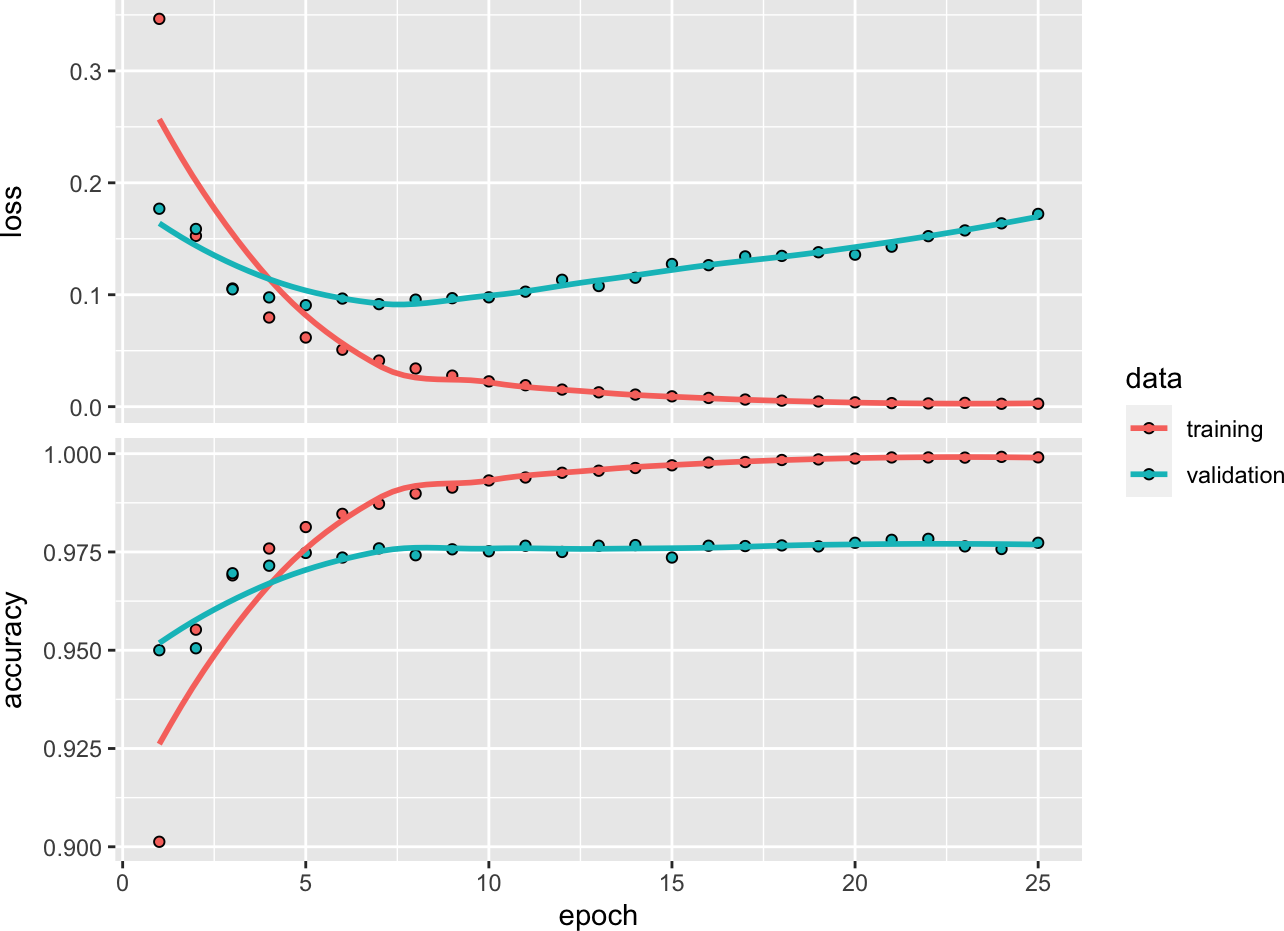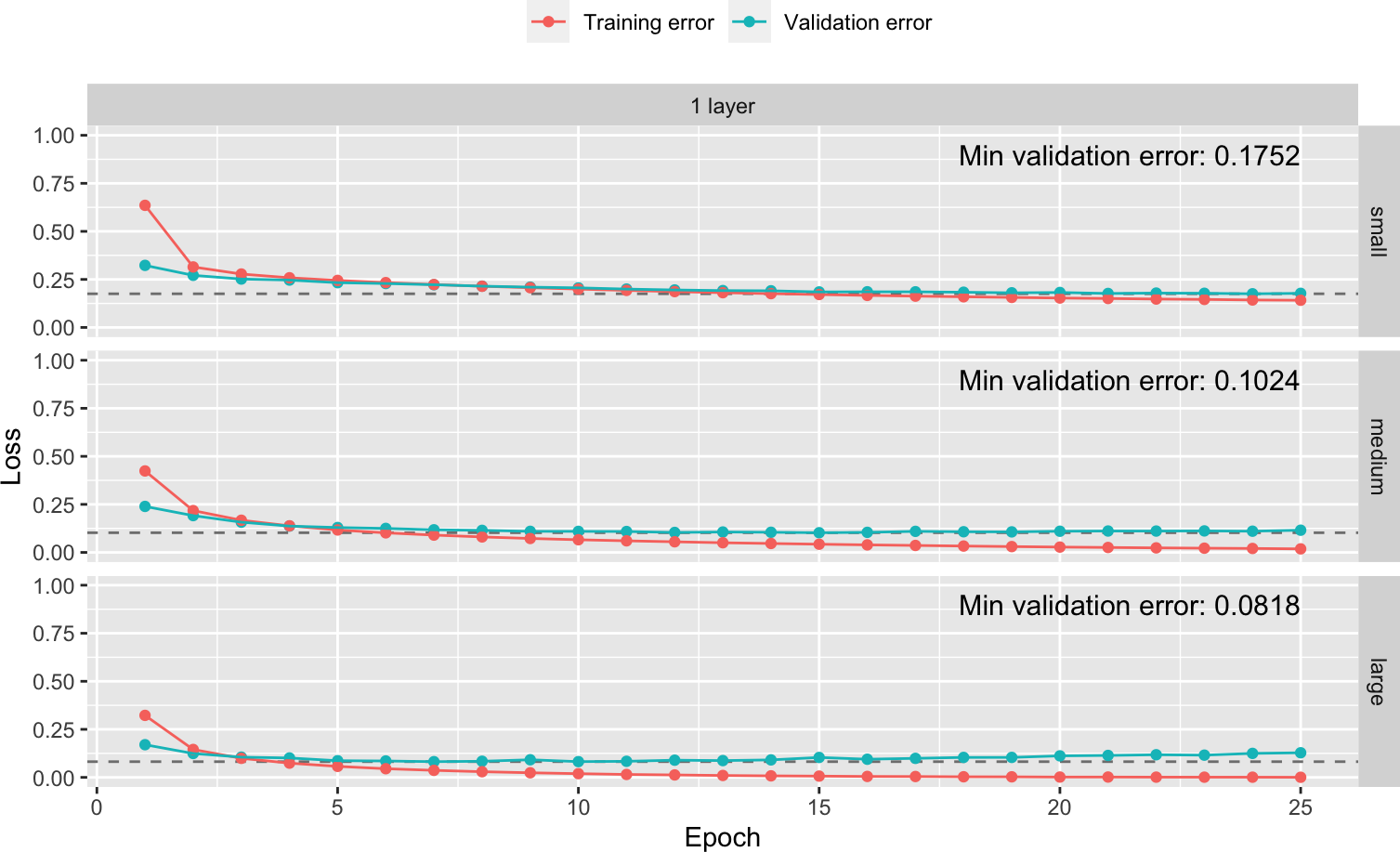# Contents

$$\newcommand{\vect}{\boldsymbol{#1}}$$ $$\newcommand{\transp}{^{\text{T}}}$$ $$\newcommand{\mat}{\boldsymbol{\mathcal{#1}}}$$ $$\newcommand{\sign}{\text{sign}}$$

# 1 Goals

In this tutorial, we mainly use the MNIST dataset to explore classification deep neural networks (DNN) models. At the end of this tutorial, you should be comfortable to use a software package (here keras) to run different models for a classification task. You will explore different models by exploring/tuning different hyperparamaters of the DNN:

• number of layers and nodes
• batch normalization
• regularization technique
• dropout
• weight initialization

# 2 MNIST Data set

First you have to install keras R packages using

install.packages(keras)

We load some extra packages that you have to install too.

library(dplyr)         

Attaching package: 'dplyr'
The following objects are masked from 'package:stats':

filter, lag
The following objects are masked from 'package:base':

intersect, setdiff, setequal, union
library(keras)
library(dslabs)
library(tidyr)
library(stringr)
library(purrr)

MNIST data are available from the package dslabs

# Import MNIST training data
mnist_x <- mnist$train$images
mnist_y <- mnist$train$labels
mnist_x_test <- mnist$test$images
mnist_y_test <- mnist$test$labels
dim(mnist_x)
 60000   784
dim(mnist_x_test)
 10000   784
length(mnist_y)
 60000
length(mnist_y_test)
 10000

Reminder: Keep in mind that all features need to be numeric for running a feedforward DNN. When you have some categorical features you have to transform into numerical values such as one-hot encoded.

### 2.1.1 Scale the data set

The data is in gray scale with each image having 0 to 255 pixels. We therefore need to scale the data by the number of pixels.

colnames(mnist_x) <- paste0("V", 1:ncol(mnist_x))
mnist_x <- mnist_x / 255
colnames(mnist_x_test) <- paste0("V", 1:ncol(mnist_x))
mnist_x_test <- mnist_x_test / 255

### 2.1.2 Transform the outcome

For multi-classification model (multinomial response 0 to 9), Keras uses one-hot encoded for the outcome

# One-hot encode response
mnist_y <- to_categorical(mnist_y, 10)
dim(mnist_y)
 60000    10

## 2.2 Implementation a DNN using Keras

### 2.2.1 Procedure

• Initiate a sequential feed-forward DNN using keras_model_sequential()
model <- keras_model_sequential() %>%
layer_dense(units = 128, input_shape = ncol(mnist_x)) %>%
layer_dense(units = 64) %>%
layer_dense(units = 10)

Here, we have two hidden layers:

• 128 neurons for the first layer
• 64 for the second
• 10 neurons for the output layer

Note the input_shape argument which represents the number of features in the data (here 784)

### 2.2.2 Activation

We need to choose some activation function:

model <- keras_model_sequential() %>%
layer_dense(units = 128, activation = "relu", input_shape = ncol(mnist_x)) %>%
layer_dense(units = 64, activation = "relu") %>%
layer_dense(units = 10, activation = "softmax")

Here, it is natural to choose the softmax function for the output layer. It is the most common to use ReLU activation for hidden layer

### 2.2.3 Backpropagation and Optimizer

Now, we have to define our objective function to optimize and the optimizer to get a solution. The natural choise here is the cross entropy for categorical outcome. You have learned in lecture 3 the different variant of the gradient descent. Keras offers several optimizers:

• Stochastic gradient descent (sgd) optimizer
• rmsprop

We will use in this example rmsprop optimizer.

model <- keras_model_sequential() %>%

# Network architecture
layer_dense(units = 128, activation = "relu", input_shape = ncol(mnist_x)) %>%
layer_dense(units = 64, activation = "relu") %>%
layer_dense(units = 10, activation = "softmax") %>%

# Backpropagation
compile(
loss = 'categorical_crossentropy',
optimizer = optimizer_rmsprop(),
metrics = c('accuracy')
)

Note that we define a metric on which the model will be evaluated. We have used the “accuracy” metric to assess the performance of the model

### 2.2.4 Summary of our modef

summary(model)
Model: "sequential_2"
________________________________________________________________________________
Layer (type)                        Output Shape                    Param #
================================================================================
dense_8 (Dense)                     (None, 128)                     100480
________________________________________________________________________________
dense_7 (Dense)                     (None, 64)                      8256
________________________________________________________________________________
dense_6 (Dense)                     (None, 10)                      650
================================================================================
Total params: 109,386
Trainable params: 109,386
Non-trainable params: 0
________________________________________________________________________________

### 2.2.5 Train our model

We will train our model on 25 epochs and a bath size of 128. We also use $$20\%$$ of our data for the evaluation step during the training phase, meaning that $$60,000\times 0.2=12,000$$ of the samples are using for the validation step while 48,000 samples are using for the optimization step.

Reminder: An epoch describes the number of times the algorithm sees the entire data set. So with a batch size of 128, one epoch is achieved after 375 passes.

# Train the model
set.seed(1)
fit1 <- model %>%
fit(
x = mnist_x,
y = mnist_y,
epochs = 25,
batch_size = 128,
validation_split = 0.2,
verbose = FALSE
)

# Display output
fit1

Final epoch (plot to see history):
loss: 0.002657
accuracy: 0.9991
val_loss: 0.1722
val_accuracy: 0.9773 
plot(fit1)
geom_smooth() using formula 'y ~ x'Figure 1: Training and validation performance over 25 epochs

We can see that the loss function improves rapidly. However, we can see a potential overfit after 10 epochs. Indeed, the accurary rate of the validation set presents a flat shape after 10 epochs.

### 2.2.6 Prediction

We can predict now the class (digits) for a new image:

model%>% predict_classes(mnist_x_test[1:10,])
  7 2 1 0 4 1 4 9 6 9

Compared to the true label

mnist_y_test[1:10]
  7 2 1 0 4 1 4 9 5 9

It looks good ?

pred <- model%>% predict_classes(mnist_x_test[,])
table(pred,mnist_y_test)
    mnist_y_test
pred    0    1    2    3    4    5    6    7    8    9
0  973    0    2    0    2    2    4    2    2    4
1    0 1114    0    0    0    1    2    2    0    1
2    0    5 1007    1    5    0    1   13    6    0
3    3    1    6  997    1    9    1    2    7   10
4    0    0    1    0  940    1    3    1    2    2
5    1    2    0    4    2  869    5    0    3    2
6    1    4    3    0    5    4  940    0    0    1
7    1    3    4    3    4    0    0 1001    4    7
8    1    6    9    3    2    3    2    3  947    5
9    0    0    0    2   21    3    0    4    3  977
sum(diag(table(pred,mnist_y_test)))/10000
 0.9765

### 2.2.7 Performance on test set

library(caret)
confusionMatrix(factor(pred),factor(mnist_y_test))->res
res$table  Reference Prediction 0 1 2 3 4 5 6 7 8 9 0 973 0 2 0 2 2 4 2 2 4 1 0 1114 0 0 0 1 2 2 0 1 2 0 5 1007 1 5 0 1 13 6 0 3 3 1 6 997 1 9 1 2 7 10 4 0 0 1 0 940 1 3 1 2 2 5 1 2 0 4 2 869 5 0 3 2 6 1 4 3 0 5 4 940 0 0 1 7 1 3 4 3 4 0 0 1001 4 7 8 1 6 9 3 2 3 2 3 947 5 9 0 0 0 2 21 3 0 4 3 977 res$overall
Accuracy
0.9765 

## 2.3 Improve our model by tuning some parameters

### 2.3.1 Model complexity

We will explore different model size by playing with the number of hidden layer from 1 to 3 and different number of neurons. Complex models have higher capacity to learn more features and patterns in the data, however they can overfit the training data. We try to maximize a high validation performance while minimizing the complexity of our model. The folowing table present the 9 models we will explore:

Table 1: 9 Models with different complexity according number of layers and nodes per layer.
Hidden Layers
Size 1 2 3
small 16 16, 8 16, 8, 4
medium 64 64, 32 64, 32, 16
large 256 256, 128 256, 128, 64

We have 9 models to run !!! better to wrap it into a nice function.

### 2.3.2 Small Model: one layer model

compiler <- function(object) {
compile(
object,
loss = 'categorical_crossentropy',
optimizer = optimizer_rmsprop(),
metrics = c('accuracy')
)
}

trainer <- function(object) {
fit(
object,
x = mnist_x,
y = mnist_y,
epochs = 25,
batch_size = 128,
validation_split = .2,
verbose = FALSE
)
}

# One layer models -------------------------------------------------------------
# small  model
1 layer_small <- keras_model_sequential() %>%
layer_dense(units = 16, activation = "relu", input_shape = ncol(mnist_x)) %>%
layer_dense(units = 10, activation = "softmax") %>%
compiler() %>%
trainer()

# medium
1 layer_medium <- keras_model_sequential() %>%
layer_dense(units = 64, activation = "relu", input_shape = ncol(mnist_x)) %>%
layer_dense(units = 10, activation = "softmax") %>%
compiler() %>%
trainer()

# large
1 layer_large <- keras_model_sequential() %>%
layer_dense(units = 256, activation = "relu", input_shape = ncol(mnist_x)) %>%
layer_dense(units = 10, activation = "softmax") %>%
compiler() %>%
trainer()

We can plot the results:

models <- ls(pattern = "layer_")
df <- models %>%
map(get) %>%
map(~ data.frame(
Validation error = .$metrics$val_loss,
Training error   = .$metrics$loss,
epoch = seq_len(.$params$epoch)
)) %>%
map2_df(models, ~ mutate(.x, model = .y)) %>%
separate(model, into = c("Middle layers", "Number of nodes"), sep = "_") %>%
gather(Validation, Loss, Validation.error:Training.error) %>%
mutate(
Validation = str_replace_all(Validation, "\\.", " "),
Number of nodes = factor(Number of nodes, levels = c("small", "medium", "large"))
)

best <- df %>%
filter(Validation == "Validation error") %>%
group_by(Middle layers, Number of nodes) %>%
filter(Loss == min(Loss)) %>%
mutate(label = paste("Min validation error:", round(Loss, 4)))

ggplot(df, aes(epoch, Loss)) +
geom_hline(data = best, aes(yintercept = Loss), lty = "dashed", color = "grey50") +
geom_text(data = best, aes(x = 25, y = 0.95, label = label), size = 4, hjust = 1, vjust = 1) +
geom_point(aes(color = Validation)) +
geom_line(aes(color = Validation)) +
facet_grid(Number of nodes ~ Middle layers, scales = "free_y") +
scale_y_continuous(limits = c(0, 1)) +
theme(legend.title = element_blank(),
legend.position = "top") +
xlab("Epoch")